# ISC Class 12 Chemistry Question Paper Solution 2017

The ISC Class 12 Chemistry – Paper I (Theory) was conducted on 20th March 2017. The exam started at 2 PM. The paper was of 70 Marks and constituted 3 hours of time duration. Students can get the ISC Class 12 Chemistry Question Paper Solution 2017 PDF. The Solution pdf provides the answers to all the questions along with the step marking for each question. Solving the previous year ISC Class 12 Chemistry Question Papers and then referring to the answers provided in the solution pdf will help students in evaluating their exam preparation. They can download the ISC Class 12 Chemistry Question Paper and Solution pdf 2017 from the link below.

Students can also access the solved ISC Class 12 Previous Year Question Papers for Maths, Chemistry, Chemistry and Biology subjects compiled at one place. They can have a look at the ISC Class 12 Chemistry Question Paper Solution 2017 below.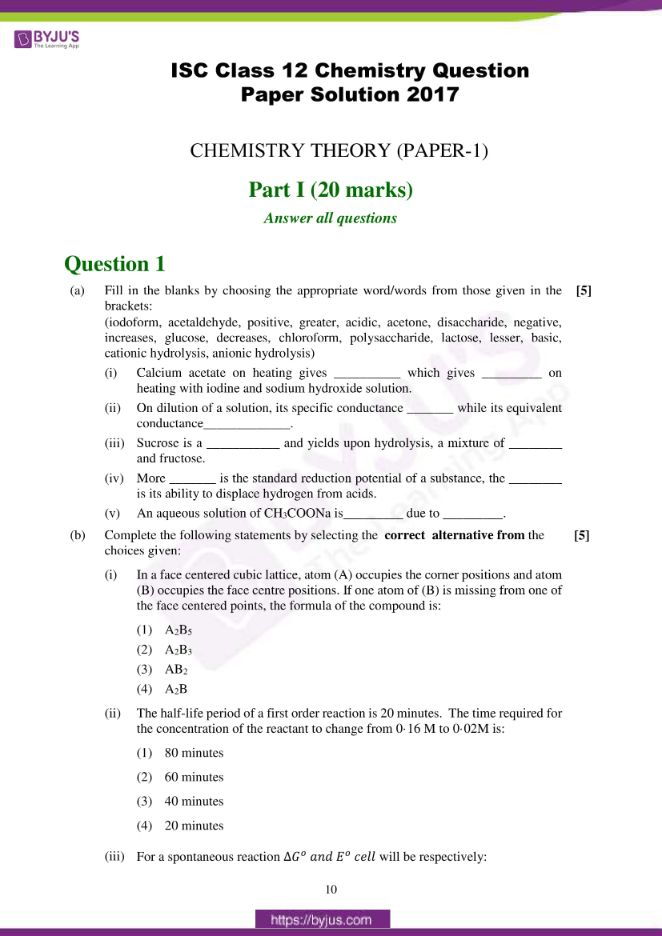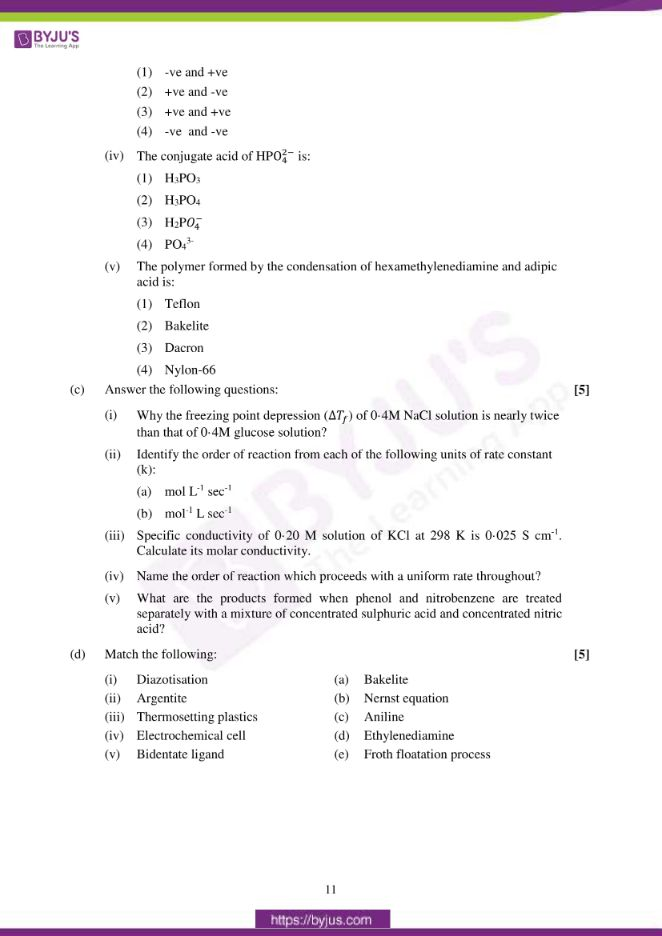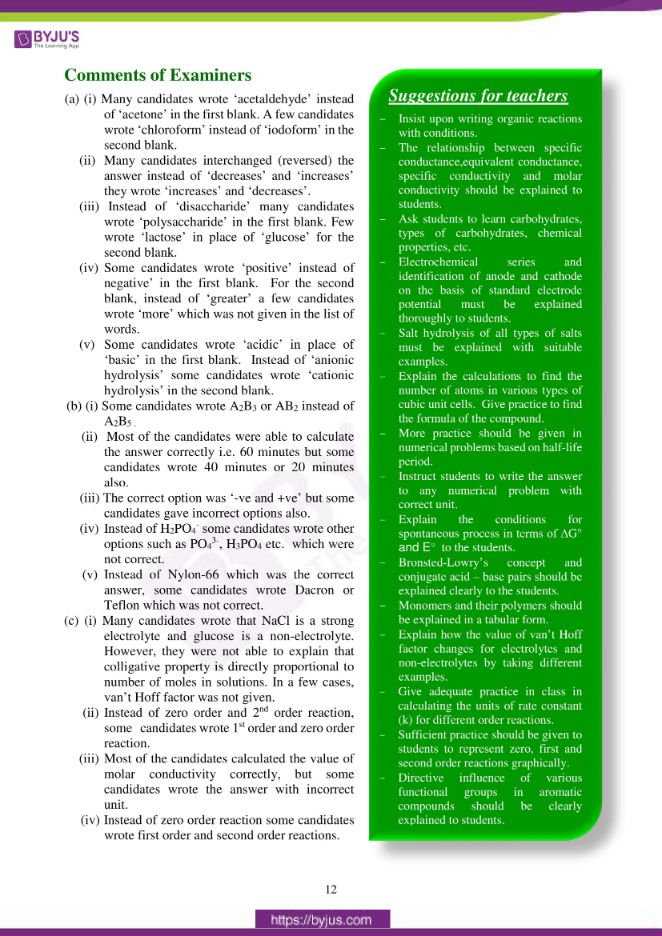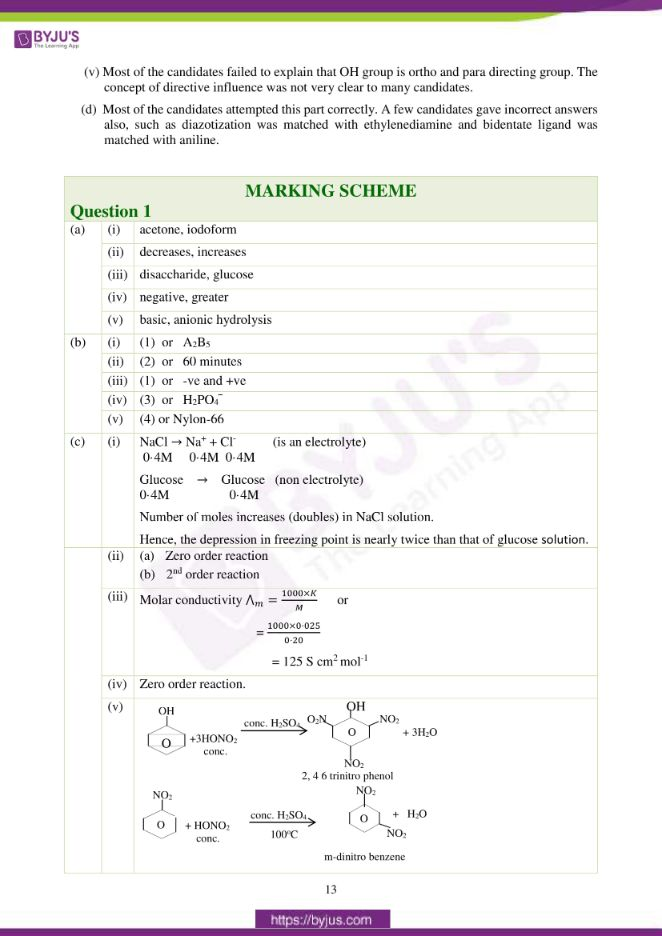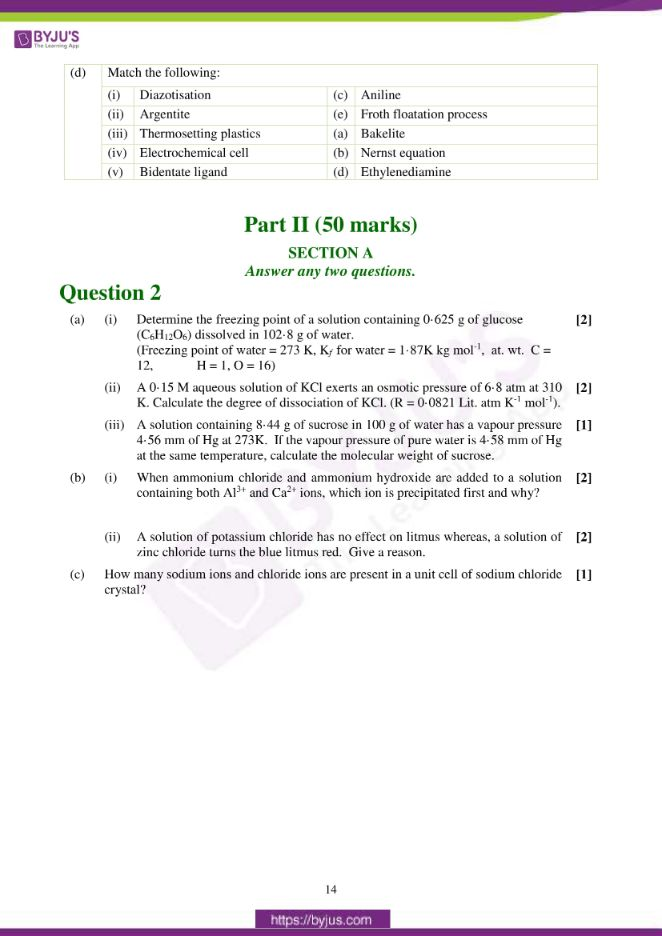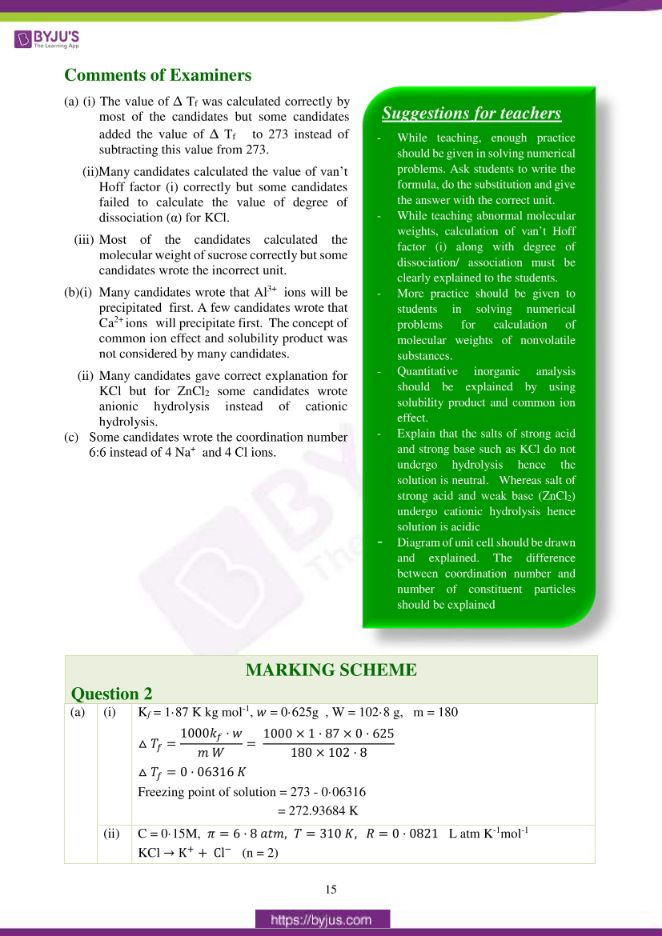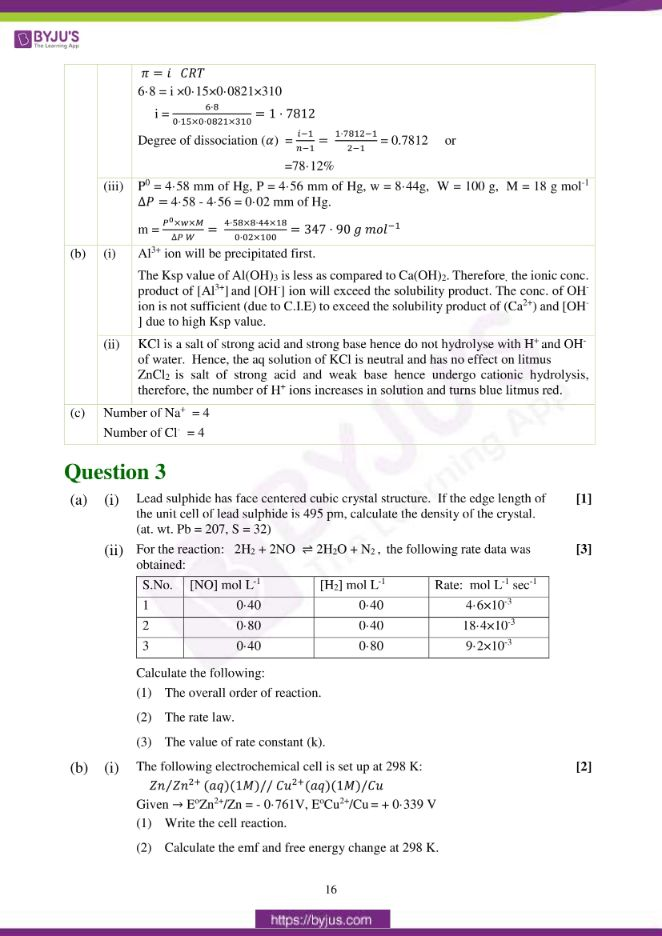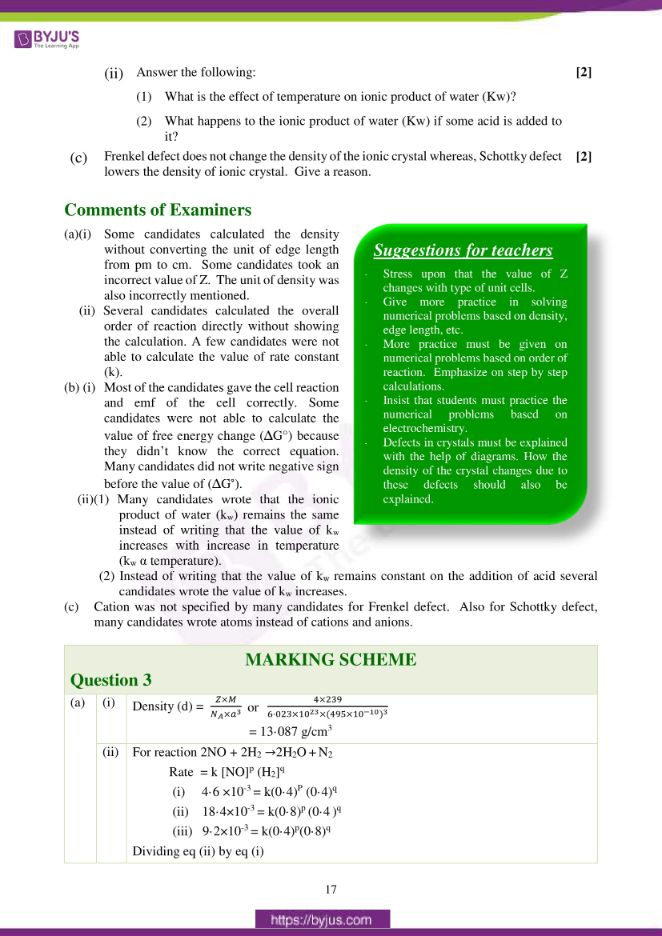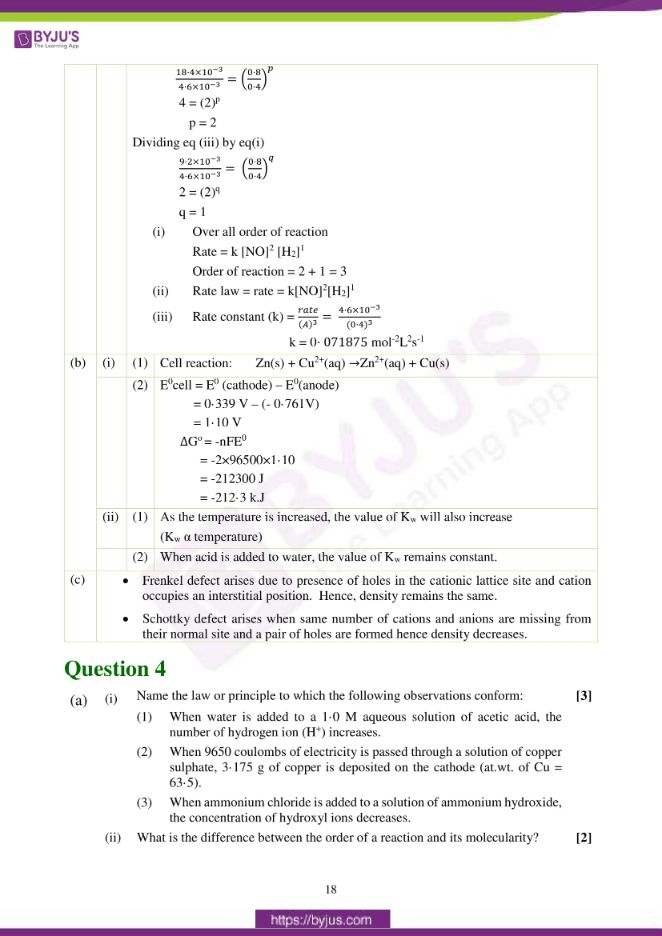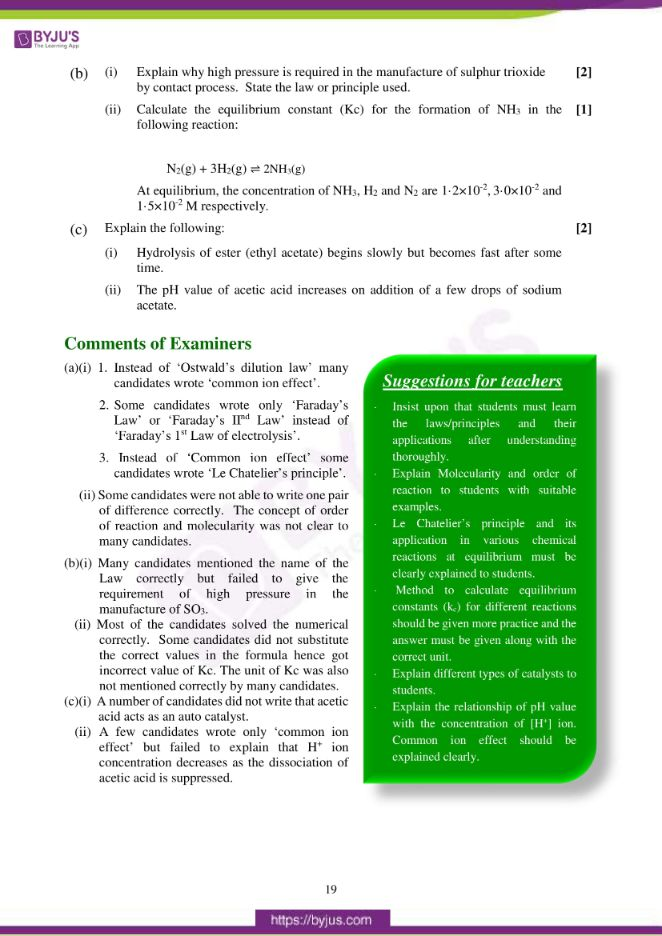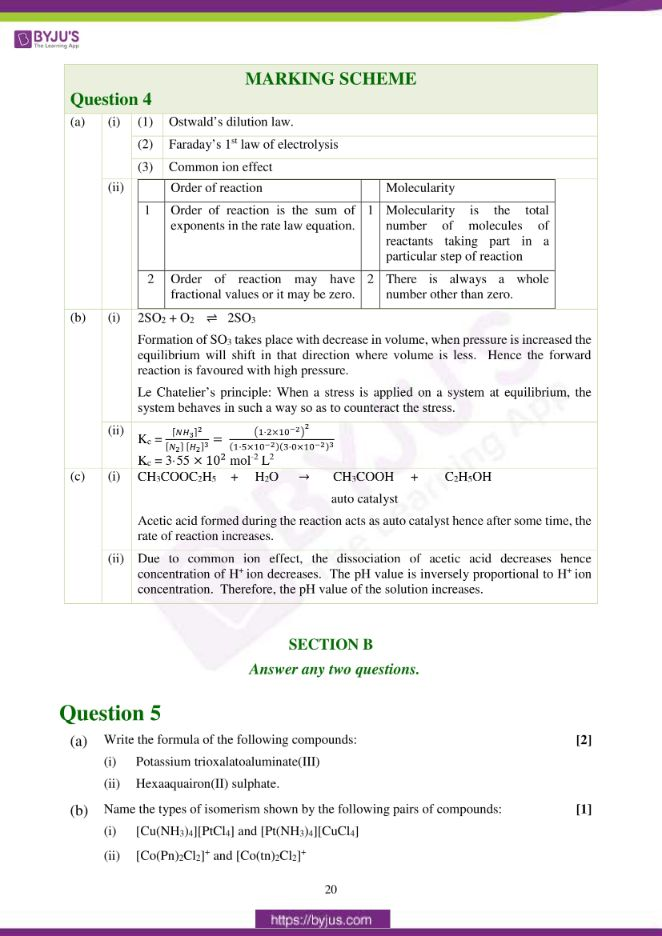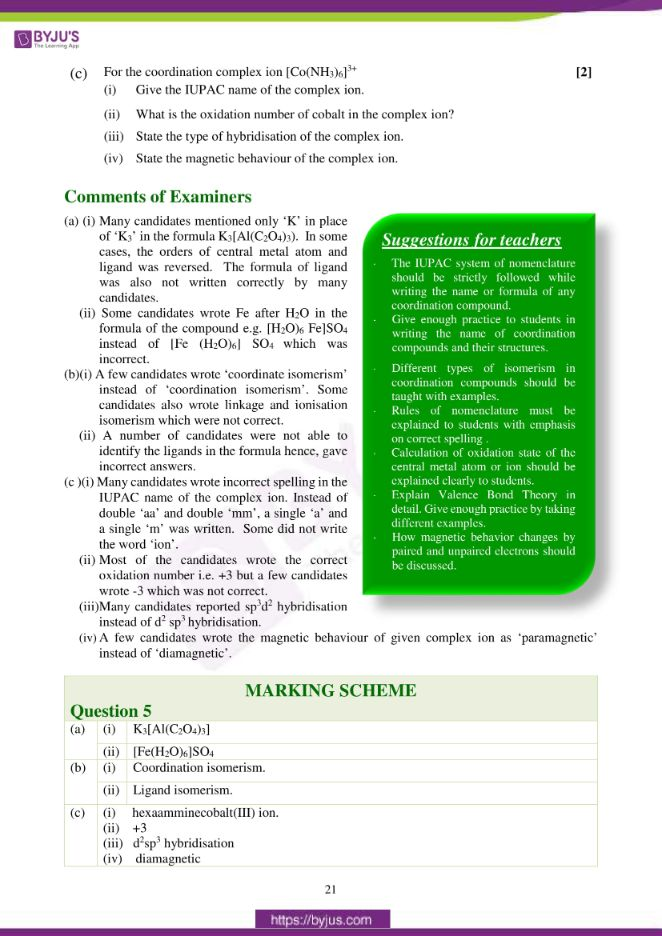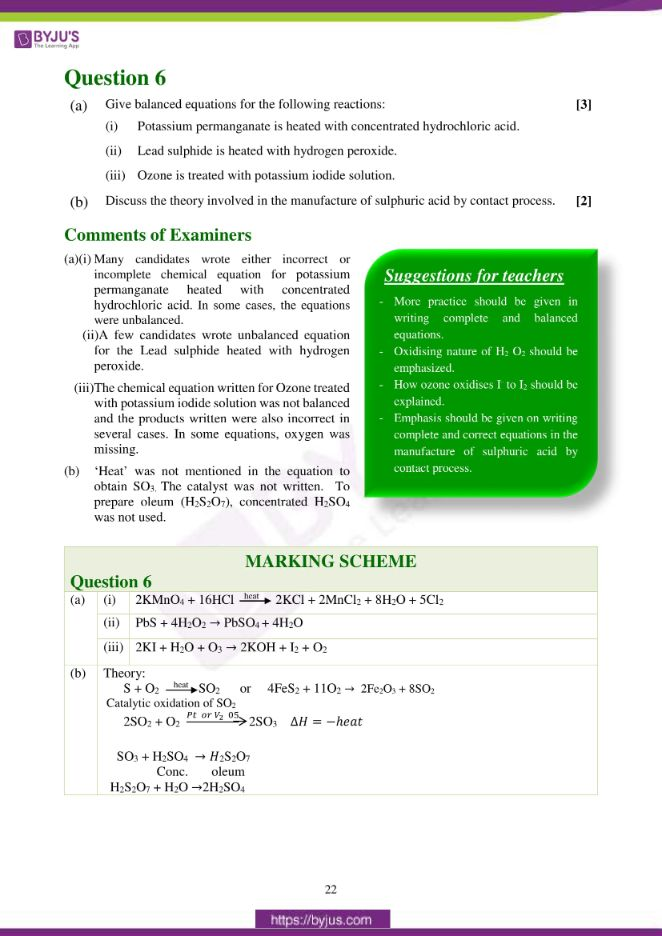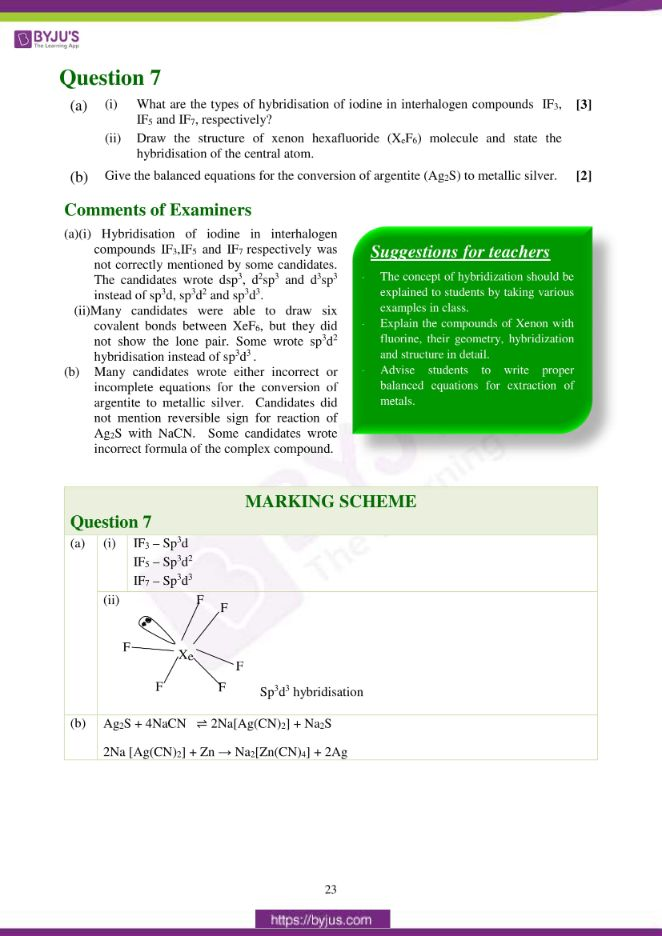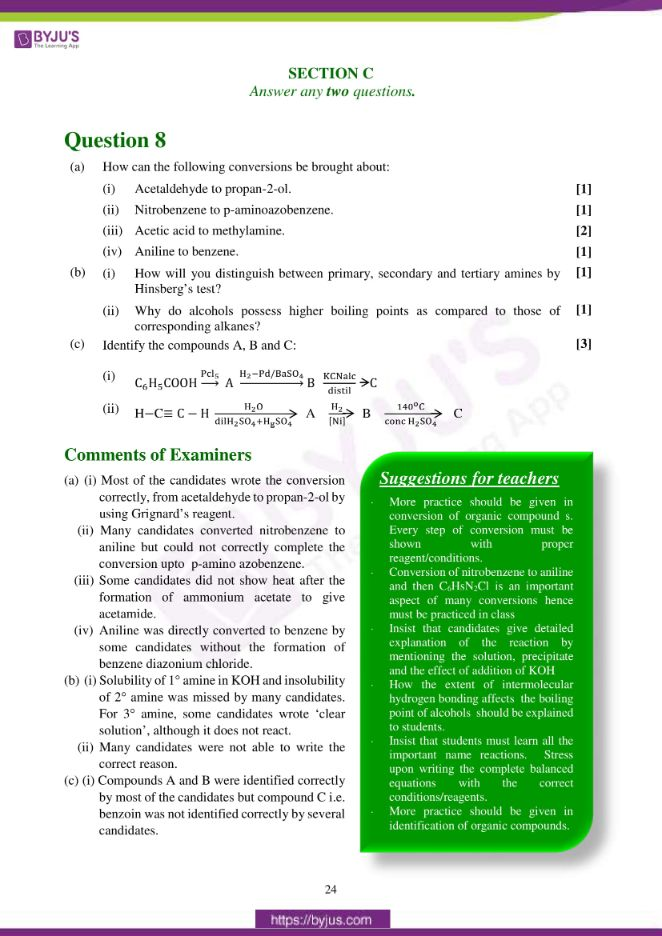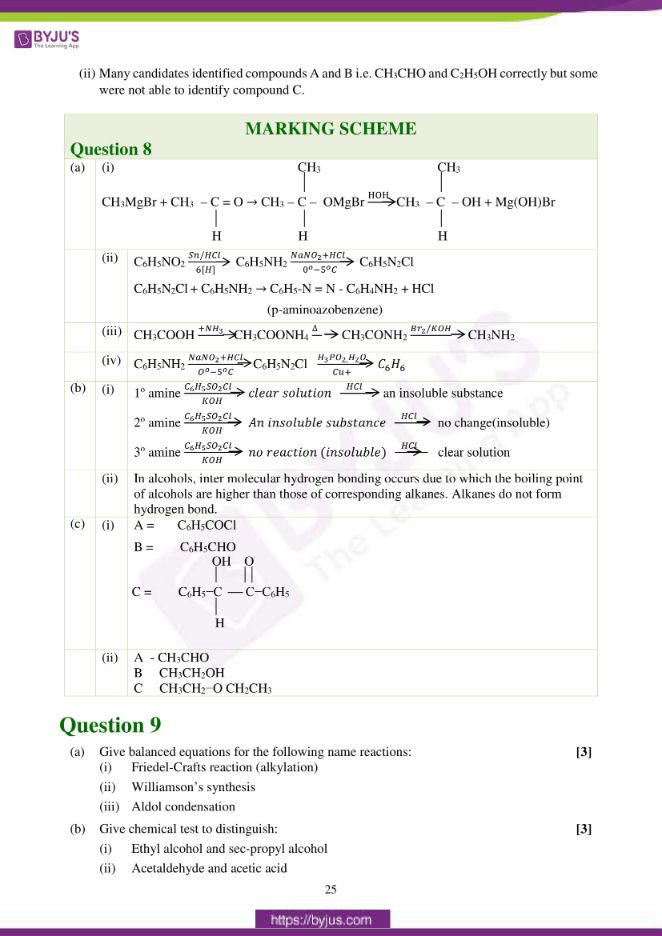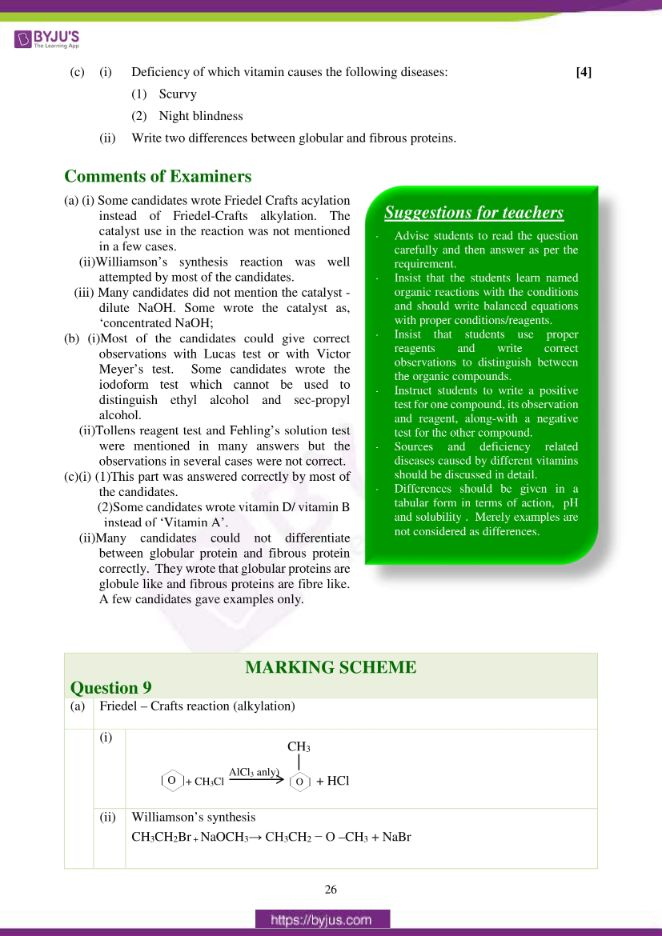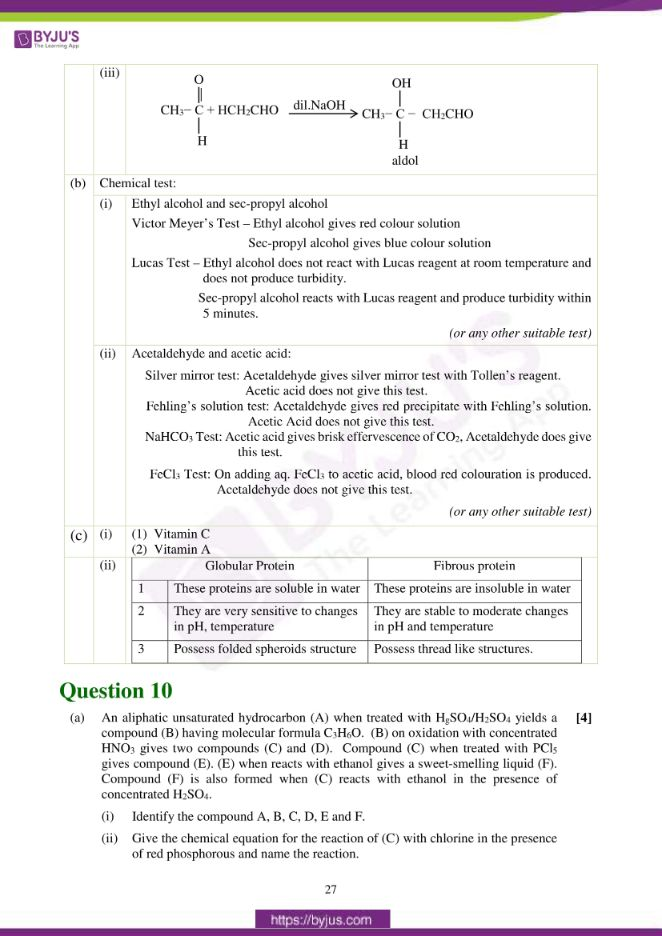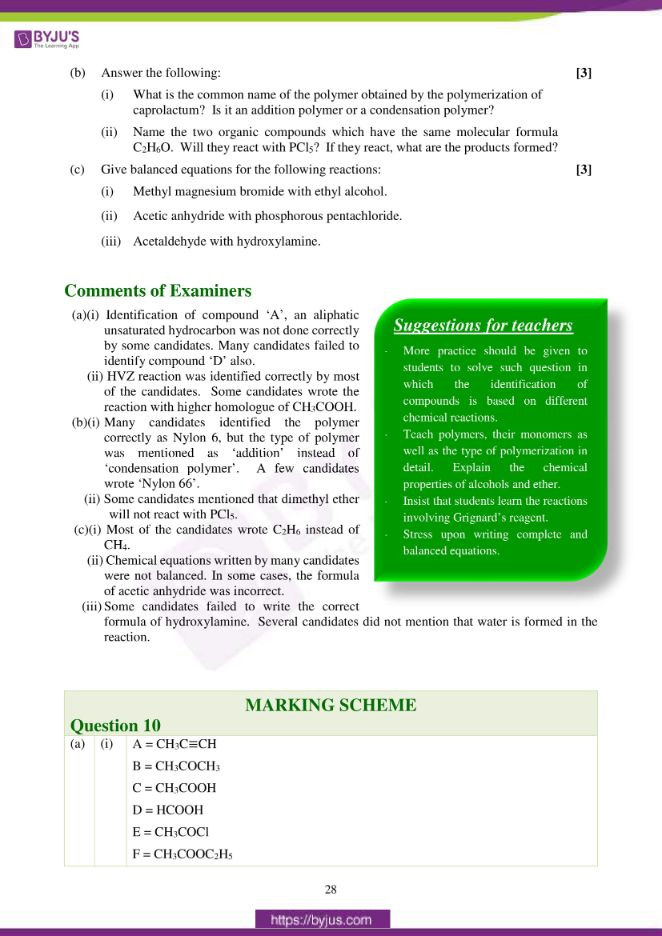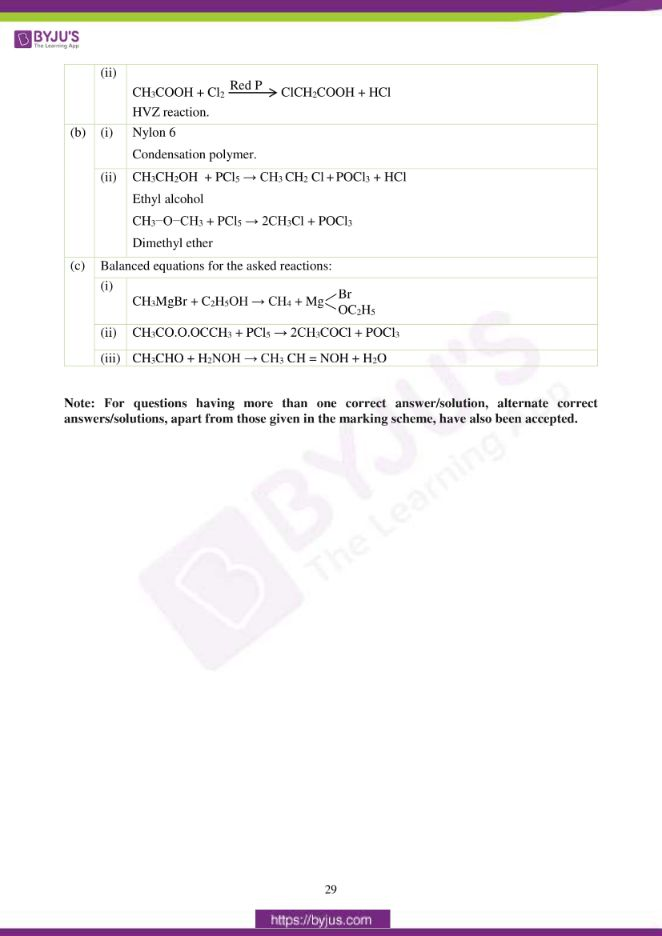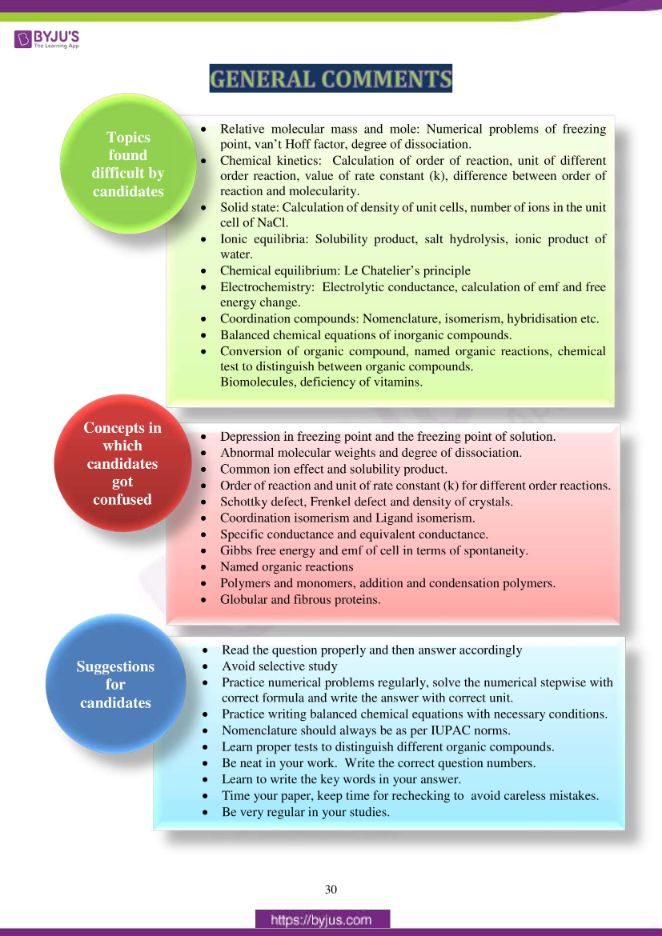### Difficult Topics of ISC Class 12 Chemistry Paper 2017

Topics which students found difficult while solving the Chemistry 2017 paper are mentioned below:

• Relative molecular mass and mole: Numerical problems of freezing point, van’t Hoff factor, degree of dissociation.
• Chemical kinetics: Calculation of order of reaction, unit of different order reaction, value of rate constant (k), difference between order of reaction and molecularity.
• Solid state: Calculation of density of unit cells, number of ions in the unit cell of NaCl.
• Ionic equilibria: Solubility product, salt hydrolysis, ionic product of water.
• Chemical equilibrium: Le Chatelier’s principle
• Electrochemistry: Electrolytic conductance, calculation of emf and free energy change. • Coordination compounds: Nomenclature, isomerism, hybridisation etc.
• Balanced chemical equations of inorganic compounds.
• Conversion of organic compounds, named organic reactions, chemical tests to distinguish between organic compounds. Biomolecules, deficiency of vitamins.

### Confusing ISC Class 12 Chemistry Questions 2017

Chemistry concepts on which students got confused during the exam are mentioned below.

• Depression in freezing point and the freezing point of solution.
• Abnormal molecular weights and degree of dissociation.
• Common ion effect and solubility product.
• Order of reaction and unit of rate constant (k) for different order reactions.
• Schottky defect, Frenkel defect and density of crystals.
• Coordination isomerism and Ligand isomerism.
• Specific conductance and equivalent conductance.
• Gibbs free energy and emf of cell in terms of spontaneity.
• Named organic reactions
• Polymers and monomers, addition and condensation polymers.
• Globular and fibrous proteins.

## ISC Class 12 Chemistry Question Paper Solution 2017

### Question 1:

(a) Fill in the blanks by choosing the appropriate word/words from those given in the

brackets:

(iodoform, acetaldehyde, positive, greater, acidic, acetone, disaccharide, negative, increases, glucose, decreases, chloroform, polysaccharide, lactose, lesser, basic, cationic hydrolysis, anionic hydrolysis)

(i) Calcium acetate on heating gives __________ which gives _________ on heating with iodine and sodium hydroxide solution.

(ii) On dilution of a solution, its specific conductance _______ while its equivalent conductance_____________.

(iii) Sucrose is a ___________ and yields upon hydrolysis, a mixture of ________ and fructose.

(iv) More _______ is the standard reduction potential of a substance, the ________ is its ability to displace hydrogen from acids.

(v) An aqueous solution of CH3COONa is_________ due to _________.

(b) Complete the following statements by selecting the correct alternative from the choices given:

(i) In a face centered cubic lattice, atom (A) occupies the corner positions and atom (B) occupies the face centre positions. If one atom of (B) is missing from one of the face centered points, the formula of the compound is:

1. A2B5
2. A2B3
3. AB2
4. A2B

(ii) The half-life period of a first order reaction is 20 minutes. The time required for the concentration of the reactant to change from 0·16 M to 0·02M is:

1. 80 minutes
2. 60 minutes
3. 40 minutes
4. 20 minutes

(iii) For a spontaneous reaction Δ𝐺𝑜 and 𝐸𝑜 will be respectively:

1. -ve and +ve
2. +ve and -ve
3. +ve and +ve
4. -ve and -ve

(iv) The conjugate acid of HPO42− is:

1. H3PO3
2. H3PO4
3. H2PO4
4. PO43-

(v) The polymer formed by the condensation of hexamethylenediamine and adipic acid is:

1. Teflon
2. Bakelite
3. Dacron
4. Nylon-66

(i) Why the freezing point depression (Δ𝑇𝑓) of 0·4M NaCl solution is nearly twice than that of 0·4M glucose solution?

(ii) Identify the order of reaction from each of the following units of rate constant (k):

1. mol L-1 sec-1
2. mol-1 L sec-1

(iii) Specific conductivity of 0·20 M solution of KCl at 298 K is 0·025 S cm-1. Calculate its molar conductivity.

(iv) Name the order of reaction which proceeds with a uniform rate throughout?

(v) What are the products formed when phenol and nitrobenzene are treated separately with a mixture of concentrated sulphuric acid and concentrated nitric acid?

(d) Match the following:

 (i) Diazotisation (a) Bakelite (ii) Argentite (b) Nernst equation (iii) Thermosetting plastics (c) Aniline (iv) Electrochemical cell (d) Ethylenediamine (v) Bidentate ligand (e) Froth floatation process

(a) (i) acetone, iodoform

(ii) decreases, increases

(iii) disaccharide, glucose

(iv) negative, greater

(v) basic, anionic hydrolysis

(b) (i) (1) or A2B5

(ii) (2) or 60 minutes

(iii) (1) or -ve and +ve

(iv) (3) or H2PO4

(v) (4) or Nylon-66

(c) (i)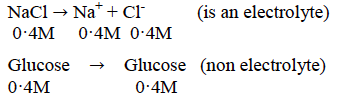Number of moles increases (doubles) in NaCl solution.

Hence, the depression in freezing point is nearly twice than that of glucose solution.

(ii)

1. Zero order reaction
2. 2nd order reaction

(iii) Molar conductivity ⋀𝑚 = 1000 × 𝐾 / 𝑀

= 1000 × 0·025 / 0·20

= 125 S cm2 mol-1

(iv) Zero order reaction.

(v)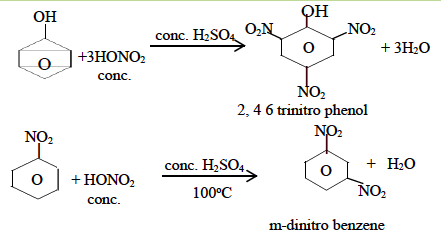(d) Match the following:

 (i) Diazotisation (c) Aniline (ii) Argentite (e) Froth floatation process (iii) Thermosetting plastics (a) Bakelite (iv) Electrochemical cell (b) Nernst equation (v) Bidentate ligand (d) Ethylenediamine

### Question 2:

(a) (i) Determine the freezing point of a solution containing 0·625 g of glucose (C6H12O6) dissolved in 102·8 g of water.

(Freezing point of water = 273 K, Kf for water = 1·87K kg mol-1, at. wt. C=12, H=1, O=16)

(ii) A 0·15 M aqueous solution of KCl exerts an osmotic pressure of 6·8 atm at 310K. Calculate the degree of dissociation of KCl. (R = 0·0821 Lit. atm K-1 mol-1).

(iii) A solution containing 8·44 g of sucrose in 100 g of water has a vapour pressure 4·56 mm of Hg at 273K. If the vapour pressure of pure water is 4·58 mm of Hg at the same temperature, calculate the molecular weight of sucrose.

(b) (i) When ammonium chloride and ammonium hydroxide are added to a solution containing both Al3+ and Ca2+ ions, which ion is precipitated first and why?

(ii) A solution of potassium chloride has no effect on litmus whereas a solution of zinc chloride turns the blue litmus red. Give a reason.

(c) How many sodium ions and chloride ions are present in a unit cell of sodium chloride

crystal?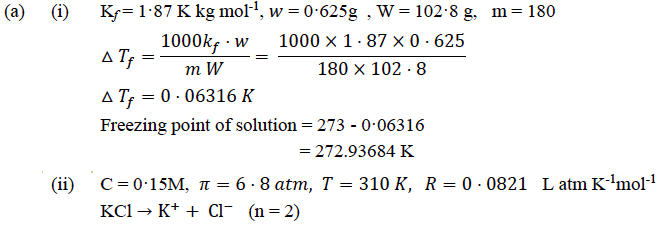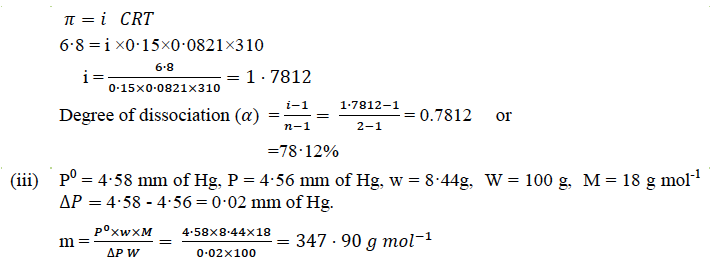(b) (i) Al3+ ion will be precipitated first.

The Ksp value of Al(OH)3 is less as compared to Ca(OH)2. Therefore, the ionic conc. product of [Al3+] and [OH] ion will exceed the solubility product. The conc. of OH ion is not sufficient (due to C.I.E) to exceed the solubility product of (Ca2+) and [OH] due to high Ksp value.

(ii) KCl is a salt of strong acid and strong base hence do not hydrolyse with H+ and OH of water. Hence, the aq solution of KCl is neutral and has no effect on litmus ZnCl2 is salt of strong acid and weak base hence undergo cationic hydrolysis, therefore, the number of H+ ions increases in solution and turns blue litmus red.

(c) Number of Na+ = 4

Number of Cl = 4

### Question 3:

(a) (i) Lead sulphide has face centered cubic crystal structure. If the edge length of the unit cell of lead sulphide is 495 pm, calculate the density of the crystal. (at. wt. Pb = 207, S = 32)

(ii) For the reaction: 2H2 + 2NO ⇌ 2H2O + N2, the following rate data was obtained:

 S.No. [NO] mol L-1 [H2] mol L-1 Rate: mol L-1 sec-1 1 0.40 0.40 4.6 × 10-3 2 0.80 0.40 18.4 × 10-3 3 0.40 0.80 9.2 × 10-3

Calculate the following:

1. The overall order of reaction.
2. The rate law.
3. The value of rate constant (k).

(b) (i) The following electrochemical cell is set up at 298 K:

Zn / Zn2+(aq)(1M) // Cu2+(aq)(1M) / Cu

Given → EoZn2+/Zn = – 0·761V, EoCu2+/Cu= + 0·339 V

1. Write the cell reaction.
2. Calculate the emf and free energy change at 298 K.

1. What is the effect of temperature on ionic product of water (Kw)?
2. What happens to the ionic product of water (Kw) if some acid is added to it?

(c) Frenkel defect does not change the density of the ionic crystal whereas, Schottky defect lowers the density of ionic crystal. Give a reason.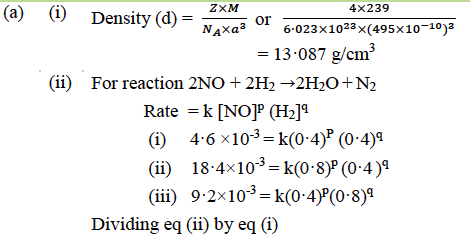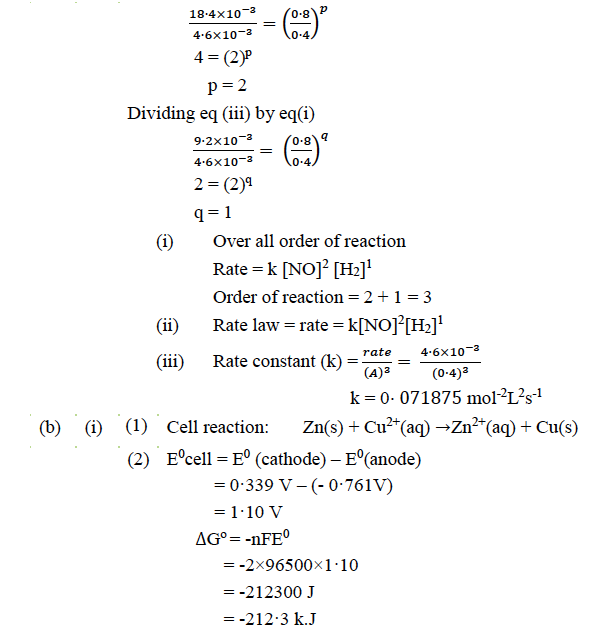(ii)

1. As the temperature is increased, the value of Kw will also increase (Kw α temperature)
2. When acid is added to water, the value of Kw remains constant.

(c)

• Frenkel defect arises due to presence of holes in the cationic lattice site and cation occupies an interstitial position. Hence, density remains the same.
• Schottky defect arises when the same number of cations and anions are missing from their normal site and a pair of holes are formed hence density decreases.

### Question 4:

(a) (i) Name the law or principle to which the following observations conform:

1. When water is added to a 1·0 M aqueous solution of acetic acid, the number of hydrogen ion (H+) increases.
2. When 9650 coulombs of electricity is passed through a solution of copper sulphate, 3·175 g of copper is deposited on the cathode (at.wt. of Cu = 63·5).
3. When ammonium chloride is added to a solution of ammonium hydroxide, the concentration of hydroxyl ions decreases.

(ii) What is the difference between the order of a reaction and its molecularity?

(b) (i) Explain why high pressure is required in the manufacture of sulphur trioxide by contact process. State the law or principle used.

(ii) Calculate the equilibrium constant (Kc) for the formation of NH3 in the following reaction:

N2(g) + 3H2(g) ⇌ 2NH3(g)

At equilibrium, the concentration of NH3, H2 and N2 are 1·2×10-2, 3·0×10-2 and 1·5×10-2 M respectively.

(c) Explain the following:

(i) Hydrolysis of ester (ethyl acetate) begins slowly but becomes fast after some time.

(ii) The pH value of acetic acid increases on addition of a few drops of sodium acetate.

1. Ostwald’s dilution law.
2. Faraday’s 1st law of electrolysis
3. Common ion effect

(ii)

 Order of reaction Molecularity Order of reaction is the sum of exponents in the rate law equation. Molecularity is the total number of molecules of reactants taking part in a particular step of reaction Order of reaction may have fractional values or it may be zero. There is always a whole number other than zero.

(b) (i) 2SO2 + O2 ⇌ 2SO3

Formation of SO3 takes place with decrease in volume, when pressure is increased the equilibrium will shift in that direction where volume is less. Hence the forward reaction is favoured with high pressure.

Le Chatelier’s principle: When a stress is applied on a system at equilibrium, the system behaves in such a way so as to counteract the stress.

(ii)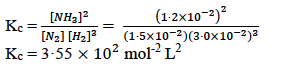(c) (i) CH3COOC2H5 + H2O → CH3COOH (auto catalyst) + C2H5OH

Acetic acid formed during the reaction acts as auto catalyst hence after some time, the rate of reaction increases.

(ii) Due to common ion effect, the dissociation of acetic acid decreases hence concentration of H+ ion decreases. The pH value is inversely proportional to H+ ion concentration. Therefore, the pH value of the solution increases.

### Question 5:

(a) Write the formula of the following compounds:

(i) Potassium trioxalatoaluminate(III)

(ii) Hexaaquairon(II) sulphate.

(b) Name the types of isomerism shown by the following pairs of compounds:

(i) [Cu(NH3)4][PtCl4] and [Pt(NH3)4][CuCl4]

(ii) [Co(Pn)2Cl2]+ and [Co(tn)2Cl2]+

(c) For the coordination complex ion [Co(NH3)6]3+

(i) Give the IUPAC name of the complex ion.

(ii)What is the oxidation number of cobalt in the complex ion?

(iii) State the type of hybridisation of the complex ion.(iv)State the magnetic behaviour of the complex ion.

(ii) [Fe(H2O)6]SO4

(b) (i) Coordination isomerism.

(ii) Ligand isomerism.

(c) (i) hexaamminecobalt(III) ion.

(ii) +3

(iii) d2sp3 hybridisation

(iv) diamagnetic

### Question 6:

(a) Give balanced equations for the following reactions:

(i) Potassium permanganate is heated with concentrated hydrochloric acid.

(ii) Lead sulphide is heated with hydrogen peroxide.

(iii) Ozone is treated with potassium iodide solution.

(b) Discuss the theory involved in the manufacture of sulphuric acid by contact process.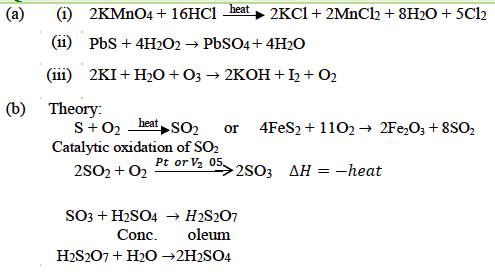### Question 7:

(a) (i) What are the types of hybridisation of iodine in interhalogen compounds IF3, IF5 and IF7, respectively?

(ii) Draw the structure of xenon hexafluoride (XeF6) molecule and state the hybridisation of the central atom.

(b) Give the balanced equations for the conversion of argentite (Ag2S) to metallic silver.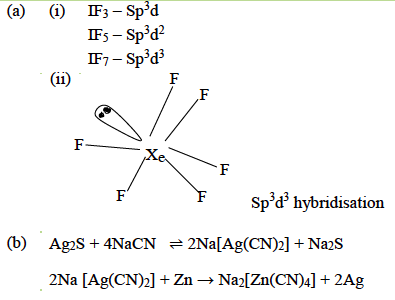### Question 8:

(a) How can the following conversions be brought about:

(i) Acetaldehyde to propan-2-ol.

(ii) Nitrobenzene to p-aminoazobenzene.

(iii) Acetic acid to methylamine.

(iv) Aniline to benzene.

(b) (i) How will you distinguish between primary, secondary and tertiary amines byHinsberg’s test?

(ii) Why do alcohols possess higher boiling points as compared to those of corresponding alkanes?

(c) Identify the compounds A, B and C: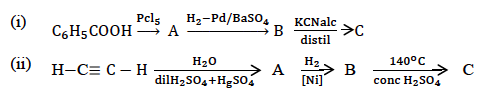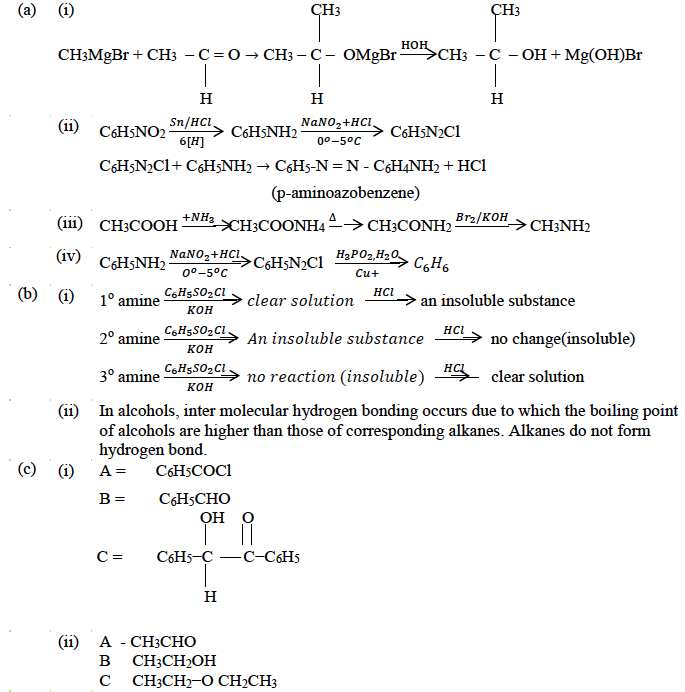### Question 9:

(a) Give balanced equations for the following name reactions:

(i) Friedel-Crafts reaction (alkylation)

(ii) Williamson’s synthesis

(iii) Aldol condensation

(b) Give chemical test to distinguish:

(i) Ethyl alcohol and sec-propyl alcohol

(ii) Acetaldehyde and acetic acid

(c) (i) Deficiency of which vitamin causes the following diseases:

1. Scurvy
2. Night blindness

(ii) Write two differences between globular and fibrous proteins.

Answer: (a) Friedel – Crafts reaction (alkylation)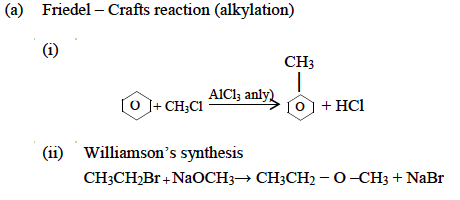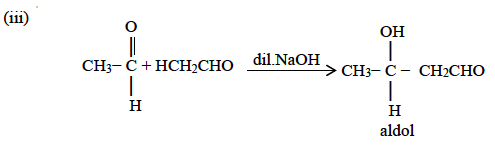(b) Chemical test:

(i) Ethyl alcohol and sec-propyl alcohol

Victor Meyer’s Test – Ethyl alcohol gives red colour solution

Sec-propyl alcohol gives blue colour solution

Lucas Test – Ethyl alcohol does not react with Lucas reagent at room temperature and does not produce turbidity.

Sec-propyl alcohol reacts with Lucas reagent and produces turbidity within 5 minutes.

(ii) Acetaldehyde and acetic acid:

Silver mirror test: Acetaldehyde gives silver mirror test with Tollen’s reagent.

Acetic acid does not give this test.

Fehling’s solution test: Acetaldehyde gives red precipitate with Fehling’s solution.

Acetic Acid does not give this test.

NaHCO3 Test: Acetic acid gives brisk effervescence of CO2, Acetaldehyde does give this test.

FeCl3 Test: On adding aq. FeCl3 to acetic acid, blood red colouration is produced.

Acetaldehyde does not give this test.

(c) (i)

1. Vitamin C
2. Vitamin A

(ii)

 Globular Protein Fibrous protein These proteins are soluble in water These proteins are insoluble in water They are very sensitive to changes in pH, temperature They are stable to moderate changes in pH and temperature Possess folded spheroids structure Possess thread like structures.

### Question 10:

(a) An aliphatic unsaturated hydrocarbon (A) when treated with HgSO4/H2SO4 yields a compound (B) having molecular formula C3H6O. (B) on oxidation with concentrated HNO3 gives two compounds (C) and (D). Compound (C) when treated with PCl5 gives compound (E). (E) when reacted with ethanol gives a sweet-smelling liquid (F). Compound (F) is also formed when (C) reacts with ethanol in the presence of concentrated H2SO4.

(i) Identify the compound A, B, C, D, E and F.

(ii) Give the chemical equation for the reaction of (C) with chlorine in the presence of red phosphorus and name the reaction.

(i) What is the common name of the polymer obtained by the polymerization of caprolactam? Is it an additional polymer or a condensation polymer?

(ii) Name the two organic compounds which have the same molecular formula C2H6O. Will they react with PCl5? If they react, what are the products formed?

(c) Give balanced equations for the following reactions:

(i) Methyl magnesium bromide with ethyl alcohol.

(ii) Acetic anhydride with phosphorus pentachloride.

(iii) Acetaldehyde with hydroxylamine.

A = CH3C≡CH

B = CH3COCH3

C = CH3COOH

D = HCOOH

E = CH3COCl

F = CH3COOC2H5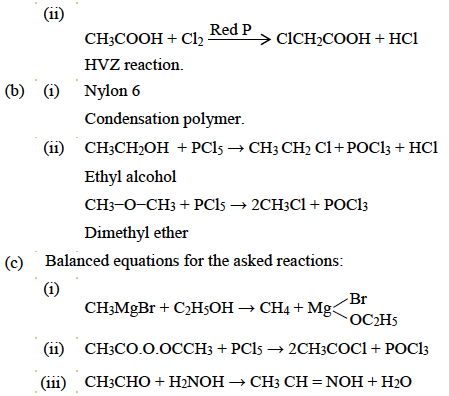We hope, solving ISC Class 12 Chemistry Question Paper Solution 2017 has boosted students’ exam preparation. Be continuous in your studies and keep practising more questions. Stay tuned to BYJU’S for the latest update on ICSE/CBSE/State Boards/Competitive exams. Also, download the BYJU’S App for interactive study videos.